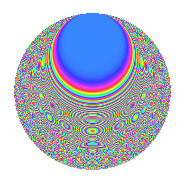# Properties

 Label 2541.2.gLevel 2541 Weight 2 Character orbit g Rep. character $$\chi_{2541}(1814,\cdot)$$ Character field $$\Q$$ Dimension 216 Sturm bound 704

# Related objects

## Defining parameters

 Level: $$N$$ = $$2541 = 3 \cdot 7 \cdot 11^{2}$$ Weight: $$k$$ = $$2$$ Character orbit: $$[\chi]$$ = 2541.g (of order $$2$$ and degree $$1$$) Character conductor: $$\operatorname{cond}(\chi)$$ = $$33$$ Character field: $$\Q$$ Sturm bound: $$704$$

## Dimensions

The following table gives the dimensions of various subspaces of $$M_{2}(2541, [\chi])$$.

Total New Old
Modular forms 376 216 160
Cusp forms 328 216 112
Eisenstein series 48 0 48

## Trace form

 $$216q + 8q^{3} + 216q^{4} - 12q^{9} + O(q^{10})$$ $$216q + 8q^{3} + 216q^{4} - 12q^{9} + 28q^{12} + 16q^{15} + 248q^{16} - 240q^{25} + 8q^{27} + 32q^{31} + 8q^{34} + 4q^{36} + 8q^{37} + 12q^{45} + 68q^{48} - 216q^{49} - 56q^{58} - 20q^{60} + 304q^{64} + 16q^{67} - 64q^{69} + 32q^{70} - 68q^{75} + 28q^{78} - 4q^{81} - 16q^{82} - 24q^{91} - 40q^{93} + O(q^{100})$$

## Decomposition of $$S_{2}^{\mathrm{new}}(2541, [\chi])$$ into newform subspaces

The newforms in this space have not yet been added to the LMFDB.

## Decomposition of $$S_{2}^{\mathrm{old}}(2541, [\chi])$$ into lower level spaces

$$S_{2}^{\mathrm{old}}(2541, [\chi]) \cong$$ $$S_{2}^{\mathrm{new}}(33, [\chi])$$$$^{\oplus 4}$$$$\oplus$$$$S_{2}^{\mathrm{new}}(231, [\chi])$$$$^{\oplus 2}$$$$\oplus$$$$S_{2}^{\mathrm{new}}(363, [\chi])$$$$^{\oplus 2}$$

## Hecke Characteristic Polynomials

There are no characteristic polynomials of Hecke operators in the database